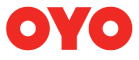New update is available. Click here to update.
Last Updated: 15 Jan, 2022

# Combination Sum IV

Moderate## Problem statement#### But if way1 =[1,2,3,4] and way2= [4,3,2,1] then both ways are different.

##### For Example:
``````If N = 3 and tar = 5 and array elements are [1,2,5] then the number of possible ways of making sum = 5 are:
(1,1,1,1,1)
(1,1,1,2)
(1,2,1,1)
(2,1,1,1)
(1,1,2,1)
(2,2,1)
(1,2,2)
(2,1,2)
(5)
Hence the output will be 9.
``````
##### Input Format :
``````The first line of the input contains an integer, 'T’, denoting the number of test cases.

The first line of each test case will contain two space-separated integers ‘N’ and “tar”, denoting the size of the array and the given integer.

The second line of each test case contains ‘N’ space-separated integers denoting elements of the array.
``````
##### Output Format :
``````For each test case, print the number of ways that satisfy the condition mentioned in the problem statement.

Print a separate line for each test case.
``````
##### Note :
``````You do not need to print anything, it has already been taken care of. Just implement the given function.
``````
##### Constraints:
``````1 <= N <= 200
1 <= nums[i] <= 1000
All the elements will be unique
1 <= tar <= 1000

Time limit: 1 sec
``````## Approaches

### 01 ApproachThe basic idea is to go over recursively to find the way such that the sum of chosen elements is “tar”. For every element, we have two choices

1. Include the element in our set of chosen elements.

2. Don’t include the element in our set of chosen elements.

When we include an element in the set then we decrease the x by the value of the element at the end. At any point, if we have x=0 then we can say that we have found the set of integers such that the sum of the set is equal to x.

Let us define a function “numberOfWays( sum, n, num)”, where “sum” is the remaining sum that we have to make, ‘n’ is the size of the array, num is the array which is given to us. Each time we have ‘n’ elements from which we have to take one.

#### Here is the algorithm:

• numberOfWays function:
• If sum is less than 0
• return 0
• If sum is 0
• Return 1
• Declare an integer variable “ans” and initialize it with 0.
• Iterate over n(say ‘j’)
• Update ans as ans + numberOfWays(sum-num[j], n, num)
• Return ans

• given function:
• Declare an integer variable n and initialize it as the size of the array.
• Return numberOfWays( tar, n, num).